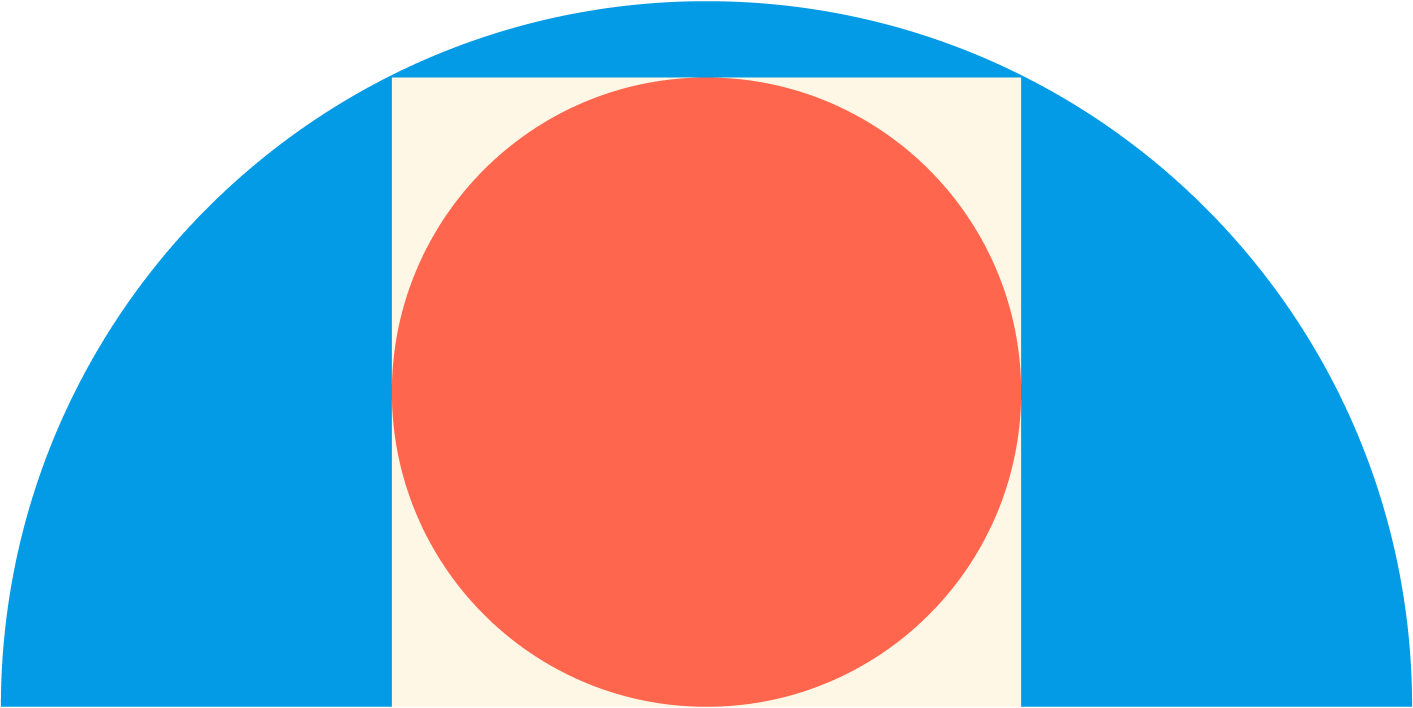# Figures Inside One Another

Geometry Level 2

The square of the figure is inscribed in a semicircle and the circle is inscribed in the square. The circle has an area of $10\text{ cm}^2$. What is the area of the semicircle?×